# Introduction

In our previous blog post, we went over the basics of the DP-SGD algorithm and introduced Opacus, a PyTorch library for training ML models with differential privacy. In this blog post, we explain how performance-improving vectorized computation is done in Opacus and why Opacus can compute “per-sample gradients” a lot faster than “microbatching” (read on to see what all these terms mean!)

# Context

Recall that differential privacy is all about worst-case guarantees, which means we need to check the gradient of each and every sample in a batch of data.

Conceptually, this is akin to writing the following PyTorch code:

While the above procedure (called the “micro batch method”, or “micro batching”) does indeed yield correct per-sample gradients, it’s grossly inefficient: GPUs really like vectorized computation, and going sample-by-sample in a for-loop entirely misses that. We acknowledged this in the ending of our last entry, leaving the explanation of how to make this faster for a later post. This is it, folks!

# Vectorized Computation

One of the features of Opacus is “vectorized computation”, in that it can compute per-sample gradients a lot faster than microbatching (they depend on the model, but we observed speedups from ~10x for small MNIST examples to ~50x for Transformers). Microbatching is simply not fast enough to run experiments and conduct research.

So, how do we do vectorized computation in Opacus? We derive the per-sample gradient formula, and implement a vectorized version of it. We will get to this soon. Let us mention that there are other methods (like this and this) that rely on computing the norm of the per-sample gradients directly. It is worth noting that since these approaches are based on computing the norm of the per-sample gradients, they do two passes of back-propagation to compute the per-sample gradients: one pass for obtaining the norm, and one pass for using the norm as a weight (see the links above for details). Although they are considered efficient, in Opacus we set out to be even more efficient (!) and do everything in one back-propagation pass.

In this blog post, we focus on the approach for efficiently computing per-sample gradients that is based on deriving the per-sample gradient formula and implementing a vectorized version of it. To make this blog post short, we focus on simple linear layers — building blocks for multi-layer perceptrons (MLPs). In our next blog post, we will talk about how we extend this approach to other layers (e.g., convolutions, LSTMs, or embeddings) in Opacus.

# Efficient Per-Sample Gradient Computation for MLP

To understand the idea for efficiently computing per-sample gradients, let’s start by talking about how AutoGrad works in the commonly-used deep learning frameworks. We’ll focus on PyTorch from now on, but to the best of our knowledge the same applies to other frameworks (with the exception of Jax).

For simplicity of explanation, we focus on one linear layer in a neural network, with weight matrix W. Also, we omit the bias from the forward pass equation: assume the forward pass is denoted by Y=WX where X is the input and Y is the output of the linear layer. If we are processing a single sample, X is a vector. On the other hand, if we are processing a batch (and that’s what we do in Opacus), X is a matrix of size d×B, with B columns (B is the batch size), where each column is an input vector of dimension d. Similarly, the output matrix Y would be of size r×B where each column is the output vector corresponding to an element in the batch and r is the output dimension.

The forward pass can be written as the following equation that captures the computation for each element in the matrix Y: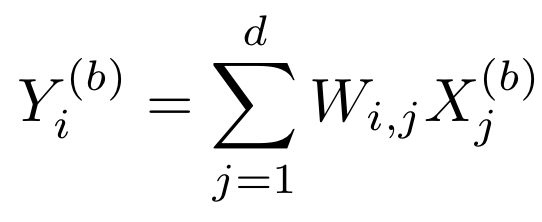Equation 1 - Forward pass of a single layer neural network

We will return to this equation shortly. Y is a matrix whose elements at row i and column b are filled in this equation (remember that the dimension of Y is r×B).

In any machine learning problem, we normally need the derivative of the loss with respect to weights W. Comparably, in Opacus we need the “per-sample” version of that, meaning, per-sample derivative of the loss with respect to weights W. Let’s first get the derivative of the loss with respect to weights, and soon, we will get to the per-sample part.

To obtain the derivative of the loss with respect to weights, we use the chain rule, whose general form is:

which can be written as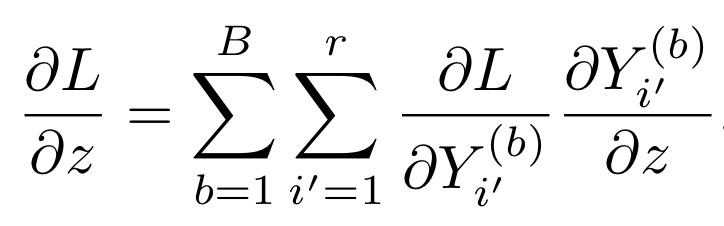Equation 3 - expanded chain rule

Now, we can replace z with Wᵢ,ⱼ and get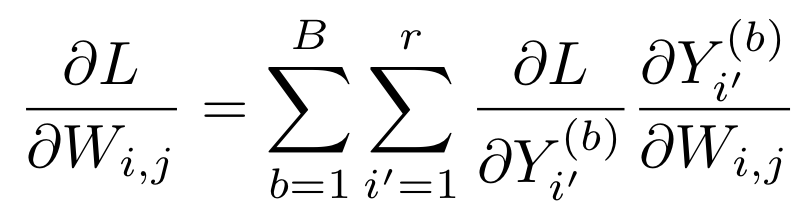Equation 4 - gradient of loss with respect to the weights

We know from the equation 1 that the second fraction in the sum (derivative of Y with respect to W) is Xⱼ⁽ᵇ⁾ when i=i’, and is 0 otherwise. Hence, we will have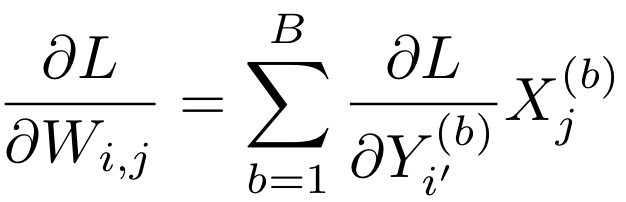Equation 5 - gradient of loss with respect to the weights (simplified)

This equation corresponds to a matrix multiplication in PyTorch.

As we can see, the gradient of loss with respect to the weight relies on the gradient of loss with respect to the output Y. In a regular backpropagation, the gradients of loss with respect to weights (or simply put, the “gradients”) are computed for the output of each layer, but they are reduced (i.e., summed up over the batch). Since Opacus requires computing per-sample gradients, what we need is the following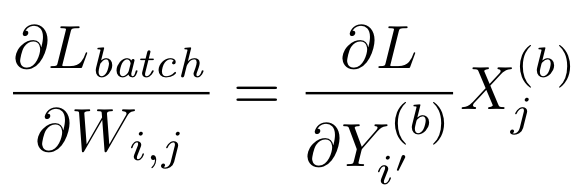Equation 6 - per-sample gradient of loss with respect to the weights

Note that these two equations are very similar; one equation has the sum over the batch and the other one does not. Let’s now focus on how we compute the per-sample gradient (this last equation) in Opacus efficiently.

A bit of notation and terminology. Recall that we used the notation Y = WX for forward pass of a single layer of a neural network. When the neural network has more layers, a better notation would be Z⁽ⁿ⁺¹⁾= W⁽ⁿ⁺¹⁾Z⁽ⁿ⁾, where n corresponds to each layer of the neural network. In that case, we can call the gradients with respect to any activations Z⁽ⁿ⁾ the “highway gradients” and the gradients with respect to the weights the “exit gradients”. They are shown in this picture.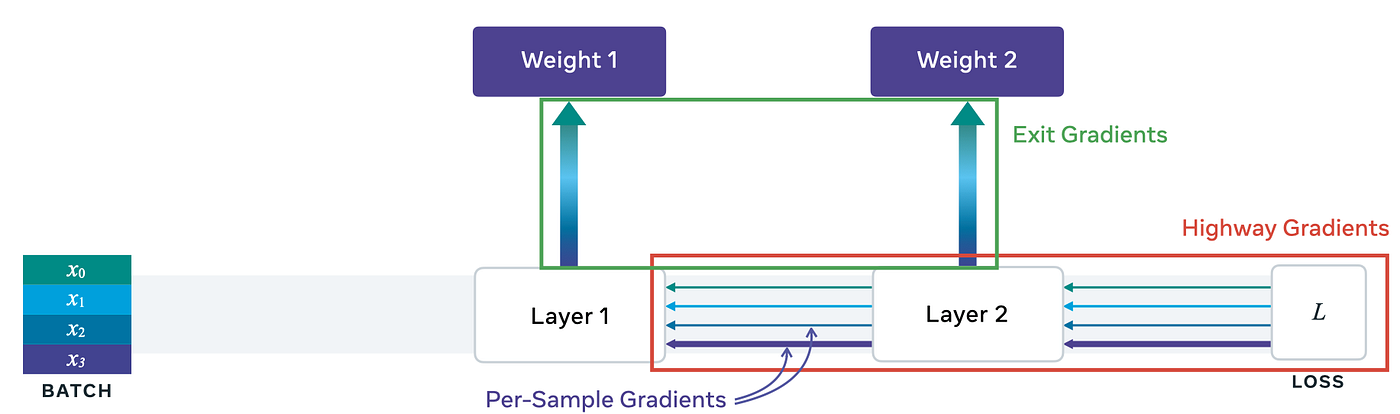Figure showing different gradients in a backpropagation for a batch of 3 data samples

If we go with this picture, explaining the issue with Autograd for computing per-sample gradients is a one-liner: highway gradients retain per-sample information, but exit gradients do not. Or, highway gradients are per-sample, but exit gradients are not necessarily. This is unfortunate because the per-sample exit gradients are exactly what we need!

So here’s the question for us:

given that we do have vectorized information in the highway, can we compute the per-sample exit gradients efficiently?

Luckily for us, there is a solution for this:

1. Store the activations somewhere.
2. Find a way to access the highway gradients.

So far, so good; but how do we store the activations and how do we access the highway gradients? Well, PyTorch has a feature to do just these: module (and tensor) hooks! Read on.

Under the hood, PyTorch is event-based and will call the hooks at the right places (your `forward` and `backward` functions are indeed being hooked where they need to go). In addition, PyTorch exposes hooks so that anyone can leverage them. The ones we care about here are these:

1. Parameter hook. This attaches to a `nn.Module`’s Parameter tensor and will always run during the backward pass. The signature is this:
`hook(grad) -> Tensor on None`

2. nn.Module hook. There are two types of these:

a. Forward hook. The signature for this is
`hook(module, input, output) -> None or modified output`

b. Backward hook. The signature for this is
`hook(module, grad_input, grad_output -> tuple(Tensor) or None`

The `grad_input` and `grad_output` are tuples that contain the gradients with respect to the inputs and outputs respectively. Read PyTorch docs about the signature of these methods here and here.

To learn more about these fundamental primitives, check out our official tutorial on hooks, or one of the excellent explainers, such as Paperspace’s or this Kaggle notebook. Finally, if you want to play with hooks more interactively, we also made a notebook for you.

We use two hooks, one forward hook and one backward hook. In the forward hook, we simply store the activations:

In the backward hook, we use the `grad_output` (highway gradient), along with the stored activations (input to the layer) to compute the per-sample gradient as below:

Now the final piece of the puzzle is the computation of the per-sample gradient itself, in the method `compute_grad_sample` above. Recall from Equation 5 that the (average) gradient of loss with respect to the weights is the result of a matrix multiplication. In order to get the per-sample gradient, we want to remove the sum reduction, as in Equation 6. This corresponds to replacing the matrix multiplication with a batched outer product. Luckily for us, torch `einsum` allows us to do that in vectorized form. The method `compute_grad_sample`is defined based on `einsum` throughout our code. For instance, for the linear layer, the main part of the code is

You can find the full implementation for the linear module here. The actual code has some bookkeeping around the `einsum` call, but the `einsum` call is the main building block of the efficient per-sample computation for us.

Since this post is already long, we refer the interested reader to read about einsum in PyTorch and do not get into the details of `einsum`. However, we really encourage you to check it out, as it’s kind of a magical thing! Just as an example, a matrix multiplication describe in

can be implemented beautifully in this line:

`c=torch.einsum('ik,kj->ij', [a, b])`

We like to highlight that `einsum` is really the key for us to have vectorized computation.

That is it folks! We just explained the last piece of the puzzle, computation of the per-sample gradient.

# Conclusion

In this blog post, we explained how vectorized computation is done in Opacus and why Opacus can compute per-sample gradients a lot faster than micro batching. We explained the idea to compute per-sample gradients efficiently for an MLP. Stay tuned for more blog posts! In our next blog post, we will talk about how we compute per-sample gradients efficiently for other layers (e.g. convolutions, LSTMs, or embeddings).

--

--## PyTorch

PyTorch is an open source machine learning platform that provides a seamless path from research prototyping to production deployment.